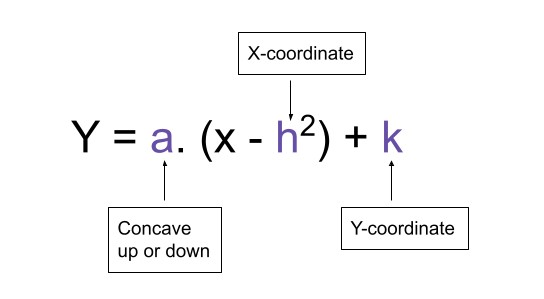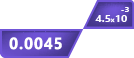# Vertex Form Calculator

Vertex form

a(x - h)² + k

## Other Calculators

This Standard form to vertex form calculator is a free tool to assist you in parabolic equations. It tells:

1. Vertex form equation
2. Standard form equation
3. Vertex
4. Y-intercept

You can find the vertex of a parabola from a vertex equation. But if you have a standard form equation then you can convert it to vertex form using this calculator.

## How to use this calculator?

To operate this calculator, you have to:

1. Choose what you want to calculate; vertex form or standard form to vertex form.
2. Enter the values.
3. Hit the calculate button.

## What is vertex form?

Quadratic equations are represented in two types of equations; Standard form and Vertex form. Both of these equations have their uses.

The vertex form is the one that gives you information about the vertex, maximum or minimum point, of a parabola. It can be derived from the standard form.

## General vertex form

A general vertex form is represented as:## How to convert the standard form into vertex form?

As mentioned before, you can convert the standard form into vertex form. A standard form is written as:

Y = ax2+bx+c

You will have to:

1. Make the coefficient of x2, 1.
2. Find the value to complete the square from the coefficient of x.
3. Close the square.
4. The equation is in standard form.

Example:

Convert y= 5x2 + 10x + 2 into vertex form.

Solution:

Step 1: Make the coefficient of x2 to be 1.

For this purpose, extract 5 from the whole equation, such as;

Y = 5 (x2 + 2x + ⅖)

Step 2: Divide the coefficient of x by 2 and take its square.

Coefficient of x = 2, so:

(2/2)2 = 12 = 1

Step 3: Add and subtract this value in the parenthesis.

Y = 5 (x2 + 2x + 1 - 1 + ⅖)

Step 4: Complete the square.

Observe that x2 + 2x + 1 = (x + 1)2 therefore,

Y = 5 (x + 1)2 - 5(1 + ⅖)

Y = 5 (x + 1)2 - 5(⅗)

Y = 5 (x + 1)2 - 3

This is the vertex form. In the last step, when we completed the square, we had to take the constant terms (i.e 1 + ⅖) aside. But since 5 was extracted from them as well, we multiplied it separately.

## How to find vertex from the quadratic equations?

You can simply find the vertex from the quadratic equations. To know how to? keep reading.

### Find vertex from the standard form:

If you don’t want to convert the standard form into the vertex form, find the vertex point using these formulas.

h = -b / (2a)

k = c - b2 / (4a)

Example:

Find the vertex of a parabola from the equation y = x2 - 3x + 1.

Solution:

The equation is in standard form so:

Step 1: Identify the elements.

a = 1

b = -3

c = 1

Step 2: Put the values in the formulas.

For h

h = -b / (2a)

h = -(-3) / 2(1)

h = 3/2 or 1.5

For k:

k = c - b2 / (4a)

k = 1 - (-3)2 /4(1)

k = - 5/4 or -1.25

So, the vertex is (3/2, -5/4). You can use these values to find the vertex form as well. Just put these values in the places of h and k while a will remain the same i.e y = 1(x + 3/2)2 - 5/4.

### Find vertex from the vertex form:

There is no rocket science in it. Simply identify the values of h and k and put them in the general vertex form equation.

Example:

What is the vertex for equation y = 9(x + 3) + 2.

Solution:

Identify the values.

h = 3

k = 2

So, the vertex is (3, 2).